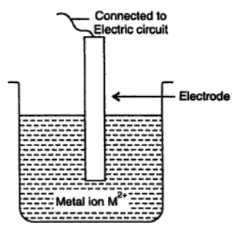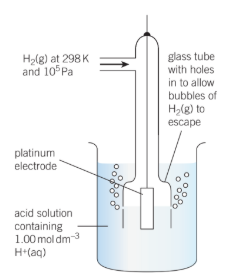# Reduction Potential

## What is Reduction Potential?

The electrode potential is oxidation potential and reduction potential termed as oxidation potential, if oxidation takes place at the electrode.Reduction involves gain of electrons, so the tendency of an electrode to gain electrons is called its reduction potential.

The equilibrium potential difference between the metal electrode and the solution surrounding it is called the electrode potential. It is also defined as the tendency of an electrode to lose or gain electrons.

### Recommended Videos on Reduction Potential## Reduction Potential Explanation

When a piece of metal is immersed in a solution of its own ions, a potential difference is created at the interface of the metal and the solution. The magnitude of the potential difference is a measure of the tendency of electrodes to undergo oxidation or reduction or tendency to lose or gain electrons.

The metal and ion represent the half cell and the reaction is half reaction. The immersed metal is an electrode and the potential due to reaction at the interface of the electrode and the solution is called the electrode potential. Thus electrode potential is the tendency of an electrode to lose or gain electrons. If the reduction takes place at the electrode, it is termed as reduction potential.If the oxidation takes place at the electrode, it is called the oxidation potential

M ⇢ M2+ + 2e

As metal ions start depositing on the metal surface this develops a positive charge on the metal rod. Since oxidation is just a reverse of reduction therefore reduction potential is obtained from the oxidation potential by simply changing the sign.

In general for an electrode

Oxidation potential = – Reduction potential

For example, in a zinc electrode the standard oxidation potential is represented as

Eo (Zn/Zn2+) = 0.76V

and standard reduction potential as

Eo (Zn2+/Zn) = -0.76V

It is a common practice to express all the electrode potentials as reduction potentials.

More recently the reduction potential has been adopted by the International Union of Pure and Applied Chemistry (IUPAC) for the designation of electrode potential.

When the half cell reaction is carried out at temperature of 298K and the electrode is suspended in a solution of one molar concentration, the electrode potential is termed as the standard electrode potential and is represented by Eo. The Standard electrode potential Eo enables one to assess the thermodynamics activity of various chemical substances. But there are no methods available by which we can measure its absolute value. The electrode potential of an electrode is measured with respect to standard hydrogen electrode.

The electrode potential of an electrode depends upon concentration of ions in solution in contact with metal. Simply oxidation potential of an electrode is inversely proportional to the concentration of ions and reduction potential is directly proportional to the concentration of ions.

## Half cells

Being a cell, a battery contains two half cells separated by an electrolyte. The electrodes are needed to connect the half cells to an external circuit. Each electrode may act as part of a redox couple, but neither has to be.

The standard conditions for the hydrogen half cell are concentration of hydrogen [H+(AQ)], pressure of hydrogen gas 105Pa and temperature 298K.

The standard hydrogen half cell is used as a reference half-cell and all other half-cells are measured against it . A list of electrode potentials has been generated relative to the standard hydrogen half-cell. The half reaction in this half cell is

2H+(aq) + 2e ⇌ H2(g)Electrodes potentials vary with temperature and so a standard temperature is defined. THis is 298K. Altering the concentration of any ions appearing in the half reactions also affects the voltages, so a standard concentration of 1.00 mol dm-3 is chosen. Standard pressure is 105Pa.

The potential of a standard hydrogen half-cell is defined as 0.0V a value chosen for convenience.

THe standard electrode potential of a half cell Eo is defined as the potential difference between the half cell and a standard hydrogen half cell.

Eo Values have a sign depending on whether the half cell is at a higher or lower positive potential than the standard hydrogen half-cell. Measurements are made at 298K with the metal dipping into a 1.00 mol dm-3 solution of a salt of the metal. Some values are

## Frequently Asked Questions on Reduction Potential

### What is the difference between oxidation potential and reduction potential?

The main distinction between the potential for oxidation and reduction is that the potential for oxidation shows a chemical element’s propensity to be oxidised. Conversely, the potential for reduction suggests the likelihood of a chemical element to be reduced.

### Is reduction potential positive or negative?

A solution with a greater (more positive) reduction potential than the new species will tend to receive electrons from the new species (i.e., to be reduced by oxidizing the new species) and a solution with a lower (more negative) reduction potential will tend to lose electrons to the new species.

### What affects reduction potential?

In general, very late transition metal ions those at the right end of the transition metal chain, including copper, silver, and gold have high potential for reduction. If the lithium’s normal reduction potential is very negative then the lithium ion’s oxidation potential is very positive.

### How do you calculate reduction potential?

The standard reduction potential can be determined by subtracting the standard reduction potential for the anode-induced reaction from the standard reduction potential for the cathode-induced reaction. The minus sign is important, since the reverse of the reduction is oxidation.

### Which element has the highest reduction potential?

Fluorine has the highest potential for decrease. High oxidizing agents prefer to oxidize other elements and are reduced by themselves. So, relative to chlorine, bromine, and iodine, fluorine has the greatest potential for reduction. High potential for reduction is due to low enthalpy of bonds and high Fluorine electronegativity.

Take up a quiz on Reduction Potential